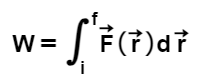# Problem: Review the work-energy theorem and apply it to a simple problem.If you push a particle of mass M in the direction in which it is already moving, you expect the particle's speed to increase. If you push with a constant force F, then the particle will accelerate with acceleration a=F/M (from Newton's 2nd law).a. Enter a one- or two-word answer that correctly completes the following statement.If the constant force is applied for a fixed interval of time t, then the _____ of the particle will increase by an amount at.b. Enter a one- or two-word answer that correctly completes the following statement.If the constant force is applied over a given distance D, along the path of the particle, then the _____ of the particle will increase by FD.  c. If the initial kinetic energy of the particle is Ki, and its final kinetic energy is Kf, express Kf in terms of Ki and the work W done on the particle.d. In general, the work done by a force F⃗  is written asNow, consider whether the following statements are true or false:The dot product assures that the integrand is always nonnegative.The dot product indicates that only the component of the force perpendicular to the path contributes to the integral.The dot product indicates that only the component of the force parallel to the path contributes to the integral.e. Assume that the particle has initial speed vi. Find its final kinetic energy Kf in terms of vi, M, F, and D.f. What is the final speed of the particle? Express your answer in terms of Kf and M.

###### FREE Expert Solution

The work-energy theorem:

$\overline{){\mathbf{∆}}{\mathbf{K}}{\mathbf{=}}{{\mathbf{W}}}_{\mathbf{n}\mathbf{e}\mathbf{t}}}$, where ΔK is the change in kinetic energy and Wnet is the net work.

a.

When a constant force is applied for a fixed time interval, the particle will accelerate.

Therefore, the speed of that particle also increases.

79% (264 ratings)###### Problem Details

Review the work-energy theorem and apply it to a simple problem.

If you push a particle of mass M in the direction in which it is already moving, you expect the particle's speed to increase. If you push with a constant force F, then the particle will accelerate with acceleration a=F/M (from Newton's 2nd law).

a. Enter a one- or two-word answer that correctly completes the following statement.

If the constant force is applied for a fixed interval of time t, then the _____ of the particle will increase by an amount at.

b. Enter a one- or two-word answer that correctly completes the following statement.

If the constant force is applied over a given distance D, along the path of the particle, then the _____ of the particle will increase by FD.

c. If the initial kinetic energy of the particle is Ki, and its final kinetic energy is Kf, express Kf in terms of Ki and the work W done on the particle.

d. In general, the work done by a force F⃗  is written asNow, consider whether the following statements are true or false:

The dot product assures that the integrand is always nonnegative.

The dot product indicates that only the component of the force perpendicular to the path contributes to the integral.

The dot product indicates that only the component of the force parallel to the path contributes to the integral.

e. Assume that the particle has initial speed vi. Find its final kinetic energy Kf in terms of vi, M, F, and D.

f. What is the final speed of the particle? Express your answer in terms of Kf and M.